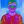0%
0%

# Arduino Battery Tester

The Arduino battery tester is a tool by which you can check how much charge a battery is.

Similar projects worth following
273 views
The project describes how much a battery is in charged with the help of an Arduino battery tester.

About Project

The Zener diode is used to test batteries that have a voltage greater than 8v.

The 2.2k ohm resistor minimizes the current coming from the battery to something that the Arduino will be able to take in.High current may damage Arduino.

The circuit system also has three various LEDs, each of these LEDs shows how much charge there is left in the battery.

• Red will shows the battery is low/almost dead.
• Yellow will presents the battery being roughly half used up.
• The green will shows the battery being full.

We connect a 100-ohm resistor to each LED individually from the ground pin to the ground connection.

Circuit connection steps are mentioned below:

1. Wire the ground pin on the Arduino to the ground rail on the breadboard.

2. Put the three LED's respectively on breadboard and connect ground pins to ground rail.

3. Locate a 100-ohm resistor onto positive end of the LEDS then connect a wire from a resistor to the relevant pins on the Arduino.

The LEDs should connect to the relevant pin numbers as mentioned below:

• Red LED = 4
• Yellow LED = 3
• Green LED = 2

4. Now connect from analogue pin 0 (A0) to the breadboard. After this add a 2.2k resistor and the Zener diode. Connect a wire from other end of diode to the ground rail.

After setting all the connection and code, your project is ready for the testing.

Internet of Things Course Training is the way to implement your ideas and skills on various applications and projects.

## Schematics

### Circuit Schematic

• 1 × Arduino Uno
• 1 × Green LED
• 1 × Red LED
• 1 × Yellow LED
• 1 × Registor 100ohm Resistor Networks / Thick Film Networks
• 1
Run a Program

int greenLed = 2;
int yellowLed = 3;
int redLed = 4;

int analogValue = 0; // analogValue variable is where we will be storing the value that comes from the analog input//
float voltage = 0;
int ledDelay = 1000; //ledDelay is how long you want the LEDs to remain on before switching off//

//set up all our LED pins as outputs//

void setup()
{
pinMode(greenLed, OUTPUT);
pinMode(yellowLed,OUTPUT);
pinMode(redLed,OUTPUT);
}

// Read the analog pin //

void loop()
{
analogValue = analogRead(A0);
voltage = 0.0048*analogValue;

//Compare calculated voltage with defined voltage values//

if( voltage >= 1.6 )
digitalWrite(greenLed, HIGH);
else if (voltage > 1.2 && voltage < 1.6)
digitalWrite(yellowLed, HIGH);
else if( voltage <= 1.2)

digitalwrite(redled, HIGH);

delay(leddelay);

digitalWrite(redLed, LOW);

digitalWrite(yellowLed, LOW);

digitalWrite(greenLed, LOW);

}

Share

## Similar Projects

Project Owner Contributor

### Attiny85, 12 LED heart soldering exerciseJan

# Does this project spark your interest?

Become a member to follow this project and never miss any updates